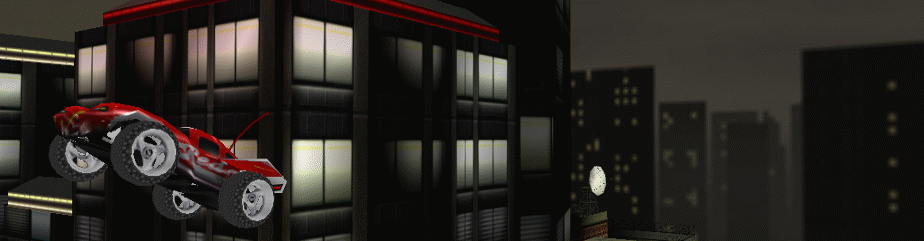Re-Volt I/O

# Car Aerial

Edit
Date: January 9, 2017
Authors: Gel38
Categories: Parameters
Tags: car_making``````;====================
; Car Aerial details
;====================

AERIAL { ; Start Aerial
SecModelNum 17
TopModelNum 18
Offset -10.000000 -22.000000 -30.000000
Direction 0.000000 -1.000000 0.000000
Length 35.000000
Stiffness 2000.000000
Damping 5.500000
} ; End Aerial``````

### 1. SecModelNum

This corresponds with the "Model Filenames" section. This setting uses the numbers -1 to 18 and determines which model is used. If no models are used, this number should be set to -1, otherwise from 0 to 18. In this case you want the number which corresponds to the vertical part of the antenna/aerial (typically number 17).

### 2. TopModelNum

This corresponds with the "Model Filenames" section. This setting uses the numbers -1 to 18 and determines which model is used. If no models are used, this number should be set to -1, otherwise from 0 to 18. In this case you want the number which corresponds to the top part of the antenna/aerial (typically number 18).

### 3. Offset

These numbers determine the placement of your 3d aerial model. It uses an XYZ coordinate system.

X-0, Z-0, Y-0

X = width of car (This is the 1st number) Y = length of car (The 3rd number) Z = height of car(2nd number)

Positive numbers on the X axis place the model to the Right, whereas, Negative numbers place it to the Left.

Positive numbers on the Z axis will move the model down toward the ground, whereas, Negative numbers will bring it up.

Positive numbers on the Y axis move the model forward, whereas, Negative numbers it to the rear

### 4. Direction

This is the angle of the aerial on XYZ coordinates with numbers ranging from -1 to 1. The first number is the X axis, the second is the Z axis, and lastly the third number is the Y axis.

With the X axis, positive numbers will tilt the aerial to the right, whereas negative numbers will tilt it to the left.

On the Y axis, positive numbers tilt the antenna to the front of the car, whereas, negative numbers will tilt it to the rear.

To adjust the Z axis the Y or X axis must first be adjusted. The Z axis is the rotation of the Aerial.

### 5. Length

This is the overall length of the aerial, from base to top. Increasing this number will make the aerial longer. Decreasing the number will make it shorter.

### 6. Stiffness

This is the rigidity of the aerial. It tells Re-Volt how much the aerial should be able to bend, or not bend. Increasing this number will increase the rigidity of the aerial, and decreasing the number will make it more floppy.

### 7. Damping

This refers to the speed the aerial will rebound after it has moved. Increasing the number gives a slower rebound, and is kind of like a blade of grass that has been stepped on, then a few hours later returns to it's original position. Lower numbers will cause the aerial to bounce back like a spring.